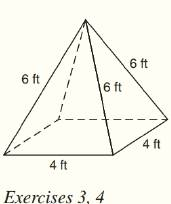Chapter 9.CT, Problem 4CT### Elementary Geometry for College St...

6th Edition
Daniel C. Alexander + 1 other
ISBN: 9781285195698

#### Solutions

Chapter
Section### Elementary Geometry for College St...

6th Edition
Daniel C. Alexander + 1 other
ISBN: 9781285195698
Textbook Problem
1 views

# For the regular square pyramid shown above, finda) the lateral area. _______b) the total area. __________To determine

a)

To find:

The lateral area of the regular square pyramid.

Explanation

Given:

The regular square pyramid is shown below

Formula used:

The lateral area of the regular pyramid is L=12lP where l is slant height and P is the perimeter of the base.

Calculation:

From the figure we see that the base has four edges each of 4 ft length.

Therefore the perimeter is 44=16 ft.

The triangular lateral face is an isosceles triangle as shown below

Here AD is the slant height l.

By Pythagoras theorem,

To determine

b)

To find:

The total area of the regular square pyramid.

### Still sussing out bartleby?

Check out a sample textbook solution.

See a sample solution

#### The Solution to Your Study Problems

Bartleby provides explanations to thousands of textbook problems written by our experts, many with advanced degrees!

Get Started

#### 29. If , find the following. (a) (b) (c) (d)

Mathematical Applications for the Management, Life, and Social Sciences

#### (i · j)k = k 2-k 0 (the number 0) 0 (the zero vector)

Study Guide for Stewart's Multivariable Calculus, 8th